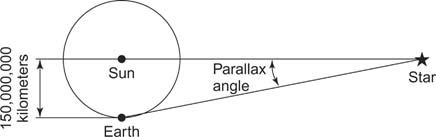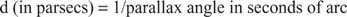## Stellar Parallax and Distances

For nearby stars, distance is determined directly from parallax by using trigonometry and the size of Earth's orbit. The trigonometric or stellar parallax angle equals one‐half the angle defined by a baseline that is the diameter of Earth's orbit. Because even the nearest stars are extremely distant, the parallax triangle is long and skinny (see Figure 1).Figure 1
Parallax.

The relationship between the parallax angle p″ (measured in seconds of arc) and the distance d is given by d = 206,264 AU/p″; for a parallax triangle with p″ = 1″, the distance to the star would correspond to 206,264 AU. By convention, astronomers have chosen to define a unit of distance, the parsec, equivalent to 206,264 AU. The parsec, therefore, is the distance to a star if the parallax angle is one second of arc, and the parallax relation becomes the much simpler formA more familiar unit of distance is the light‐year, the distance that light travels (c = 300,000 km/s) in a year (3.16 × 10 7 seconds); one parsec is the same as 3.26 light‐years.

The nearest star, α Centauri, has a parallax angle of 0.76″. Therefore its distance is d = 1/0.76″ = 1.3 pc (4 ly). The ground‐based limit of parallax measurement accuracy is approximately 0.02 arc second, limiting determination of accurate distances to stars within 50 pc (160 ly). The European Hipparcos satellite, in orbit above the atmosphere and its blurring effects, can make measurements with much higher precision, allowing accurate distance determinations to about 1000 pc (3200 ly).

Back to Top
A18ACD436D5A3997E3DA2573E3FD792A